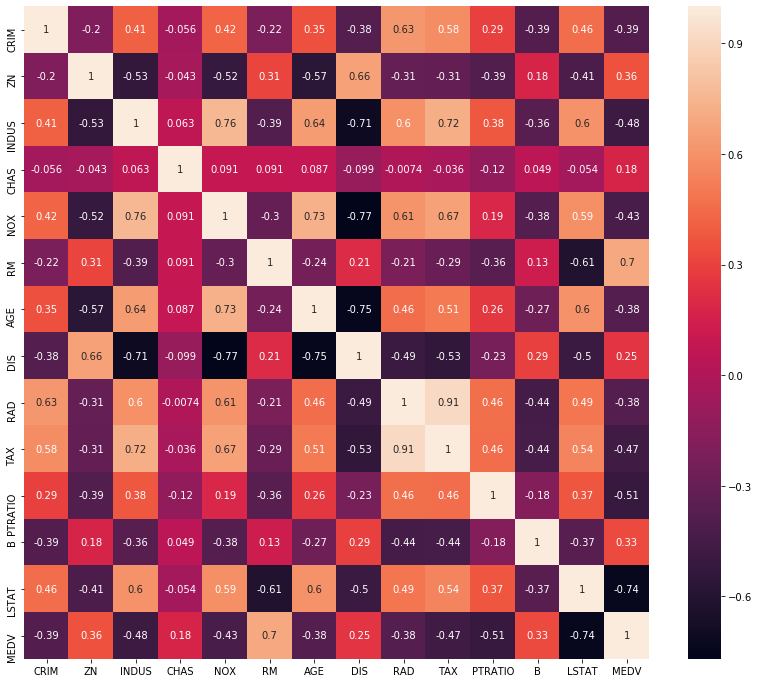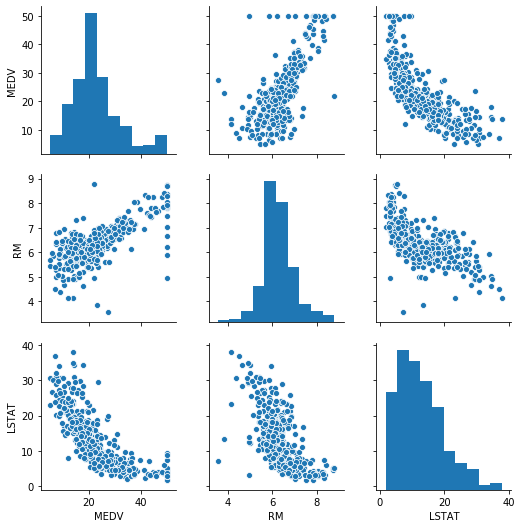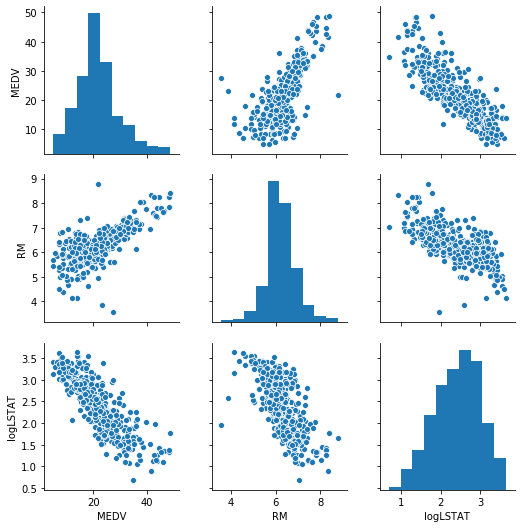# Hands-On: Supervised Learning - Linear Regression¶

### AI and Predictive Analytics in Data-Center Environments - http://dcai.bsc.es¶

In this notebook we will take a more in-depth view of the process of building a supervised learning model, particularly a Linear Regression.
We will start by importing the packages we will need for plotting and data manipulation:

In :
import numpy as np
import pandas as pd
import matplotlib.pyplot as plt
import seaborn as sns
from sklearn import datasets


For this example we will use the well-known Boston Housing dataset, which includes the median prices for houses in different neighbourhoods in the Boston area. As with the Iris dataset, Sklearn offers a pre-processed version in its datasets package so we can directly start working with it. In the same fashion as with the Iris, we can load it with the load_boston function:

In :
boston_ds = datasets.load_boston()


Sklearn also packages a description of the dataset with the structure returned by load_boston, accessble throug the DESCR attribute:

In :
print(boston_ds.DESCR)

.. _boston_dataset:

Boston house prices dataset
---------------------------

**Data Set Characteristics:**

:Number of Instances: 506

:Number of Attributes: 13 numeric/categorical predictive. Median Value (attribute 14) is usually the target.

:Attribute Information (in order):
- CRIM     per capita crime rate by town
- ZN       proportion of residential land zoned for lots over 25,000 sq.ft.
- INDUS    proportion of non-retail business acres per town
- CHAS     Charles River dummy variable (= 1 if tract bounds river; 0 otherwise)
- NOX      nitric oxides concentration (parts per 10 million)
- RM       average number of rooms per dwelling
- AGE      proportion of owner-occupied units built prior to 1940
- DIS      weighted distances to five Boston employment centres
- TAX      full-value property-tax rate per $10,000 - PTRATIO pupil-teacher ratio by town - B 1000(Bk - 0.63)^2 where Bk is the proportion of blacks by town - LSTAT % lower status of the population - MEDV Median value of owner-occupied homes in$1000's

:Missing Attribute Values: None

:Creator: Harrison, D. and Rubinfeld, D.L.

This is a copy of UCI ML housing dataset.
https://archive.ics.uci.edu/ml/machine-learning-databases/housing/

This dataset was taken from the StatLib library which is maintained at Carnegie Mellon University.

The Boston house-price data of Harrison, D. and Rubinfeld, D.L. 'Hedonic
prices and the demand for clean air', J. Environ. Economics & Management,
vol.5, 81-102, 1978.   Used in Belsley, Kuh & Welsch, 'Regression diagnostics
...', Wiley, 1980.   N.B. Various transformations are used in the table on
pages 244-261 of the latter.

The Boston house-price data has been used in many machine learning papers that address regression
problems.

.. topic:: References

- Belsley, Kuh & Welsch, 'Regression diagnostics: Identifying Influential Data and Sources of Collinearity', Wiley, 1980. 244-261.
- Quinlan,R. (1993). Combining Instance-Based and Model-Based Learning. In Proceedings on the Tenth International Conference of Machine Learning, 236-243, University of Massachusetts, Amherst. Morgan Kaufmann.



We can access the feature names through the feature_names attribute:

In :
boston_ds.feature_names

Out:
array(['CRIM', 'ZN', 'INDUS', 'CHAS', 'NOX', 'RM', 'AGE', 'DIS', 'RAD',
'TAX', 'PTRATIO', 'B', 'LSTAT'], dtype='<U7')

All the data stored in the structure returned by load_boston is in Numpy's array format, the features, labels and feature names being stored in a separate array each. This format can be somewhat inconvenient when manipulating and exploring data so in order to avoid this we will convert the dataset into Pandas' dataframe format. Pandas is Python's library for data manipulation and analysis which allows to store data in a relational database table-style with its DataFrames.

We will first create a dataframe with the features and their respective names (recall that the feature data is stored in the data attribute and the list of feature names is stored in feature_names):

In :
boston_df_raw = pd.DataFrame(data=boston_ds.data, columns=boston_ds.feature_names)


Once we have created the dataframe with the features, we can add the MEDV column containing the target values:

In :
boston_df_raw['MEDV'] = boston_ds.target


You can check the number of rows and columns of a dataframe with the shape attribute:

In :
boston_df_raw.shape

Out:
(506, 14)

You can also visualize the first rows of the dataframe with nice formatting with head. Passing a number to head will display that amount of rows, if no argument is passed it will default to displaying 5 rows:

In :
boston_df_raw.head()

Out:
CRIM ZN INDUS CHAS NOX RM AGE DIS RAD TAX PTRATIO B LSTAT MEDV
0 0.00632 18.0 2.31 0.0 0.538 6.575 65.2 4.0900 1.0 296.0 15.3 396.90 4.98 24.0
1 0.02731 0.0 7.07 0.0 0.469 6.421 78.9 4.9671 2.0 242.0 17.8 396.90 9.14 21.6
2 0.02729 0.0 7.07 0.0 0.469 7.185 61.1 4.9671 2.0 242.0 17.8 392.83 4.03 34.7
3 0.03237 0.0 2.18 0.0 0.458 6.998 45.8 6.0622 3.0 222.0 18.7 394.63 2.94 33.4
4 0.06905 0.0 2.18 0.0 0.458 7.147 54.2 6.0622 3.0 222.0 18.7 396.90 5.33 36.2

## Some preliminary analysis and data preparation¶

In this example we have a significant amount of features, and most likely not all of them will be directly related to MEDV. Because we want to avoid bloating our linear regression model with features that do not provide much (if at all) information on the target, it is advisable to first explore the data and find which ones are the features that are correlated to MEDV the most.
A particularly convenient way to do this is through a Correlation Matrix, which displays the correlations between the indicated variables. In order to visualize this in an effective way we can use a heatmapas we did with the confusion matrix in one of the previous examples:

In :
plt.figure(figsize=(14, 12))
sns.heatmap(boston_df_raw.corr(), annot=True);Cells corresponding to highly correlated variables take colors on the far ends of the scale. By analyzing the values on the heatmap above we can see that the features MEDV is correlated with the most are RM (0.7) and LSTAT (-0.74) so we will use them to make our predictions.

Another frequent way of visualizing data is through a scatterplot matrix, which allows to display the way in which different variables are related (Seaborn's version also includes the histogram for each variable on the diagonal). As we have already identified that MEDV is related to RM and LSTAT the most, we will only display the scatter matrix for those three variables:

In :
sns.pairplot(boston_df_raw[['MEDV', 'RM', 'LSTAT']]);Observing the scatterplots of MEDV against the other two variables we can see that the value 50 appears for many different different values of the other variables, and it does not follow the overall trend of the rest of the data. Because MEDV ranges from 0 to 50 these values might not correspond to an actual median price but rather be the result of truncating the real value to the maximum of the scale (50). To avoid consdering modified values we will remove them from the data:

In :
boston_df = boston_df_raw[boston_df_raw['MEDV'] < 50]


If we check the number of rows in the dataset with shape we can see we have deleted 16 rows:

In :
boston_df.shape

Out:
(490, 14)

Taking a further look to the data we can see that the relation between MEDV and LSTAT is definitely not linear. Observing LSTAT's histogram there's a clear positive skewness which suggests that we might be able to address this non-linearity by applying a logarithmic transformation. Let's create a new dataframe with the the transformed LSTAT:

In :
df = pd.DataFrame(boston_df[['MEDV', 'RM']])
df['logLSTAT'] = np.log(boston_df['LSTAT']);


And now let's plot is scatter matrix:

In :
sns.pairplot(df);Now the relationship between MEDV and logLSTAT definitely looks more like a linear one.

## Training and validation datasets¶

As we discussed in the previous notebook, we want to split our data into training and validation instances so we can test the linear regressoin model with data that has not been used to train it. For that purpose we use the train_test_split function, this time only requiring the dataframe containing both the features and label data (one of the advantages of working with dataframes):

In :
from sklearn.model_selection import train_test_split
train_df, test_df = train_test_split(df, test_size=0.3, random_state=0)


Now that the data is ready we can move on to creating and training the model. To avoid writing the feature names multiple times we can store them in a variable:

In :
feats = ['RM', 'logLSTAT']
labels = 'MEDV'


## Building the linear regression model¶

In :
from sklearn.linear_model import LinearRegression
lin_reg = LinearRegression().fit(train_df[feats].values, train_df[labels].values.reshape(-1,1))


The .values.reshape(-1,1) methods are used to reshape the numpy array into a specific shape required by Sklearn. You can see that we have also applied the fit method directly rather than creating the model and training in two separate steps.

## Evaluating the model¶

We can check the coefficients and intercept of our freshly trained model:

In :
print("Coefs: {}".format(lin_reg.coef_))
print("Intercept: {}".format(lin_reg.intercept_ ))

Coefs: [[ 2.9001909  -8.69545777]]
Intercept: [24.44541119]


The coefficients alone don't provide much insight on the quality of the model, so we can calculate the R^2 score to check how much variance on the data our model is able to explain:

In :
train_score = lin_reg.score(train_df[feats].values, train_df[labels].values.reshape(-1,1))
print("R2 Score: {}".format(train_score))

R2 Score: 0.7149388632460918


Not a great score, but take into account we are making predictions in only two variables. Let's now calculate the Root Mean Square Error:

In :
train_prediction = lin_reg.predict(train_df[feats].values)
from sklearn.metrics import mean_squared_error
rmse = np.sqrt(mean_squared_error(train_df[labels].values.reshape(-1,1), train_prediction))
print("RMSE on test data: {}".format(rmse))

RMSE on test data: 3.978592909011344


The results above are on training data, at this point in the course you might have an intuition that testing on training data alone does not give much insight on the quality of the model. To see an actual measure of the quality of our model we need to test it on the validation data. For that purpose we will predict MEDV for each one of the observations on the validation dataset and calculate the Root Mean Square Error (RMSE) with regards to the real values:

In :
test_prediction = lin_reg.predict(test_df[feats].values)
rmse = np.sqrt(mean_squared_error(test_df[labels].values.reshape(-1,1), test_prediction))
print("RMSE on test data: {}".format(rmse))

RMSE on test data: 4.734323703976905


As expected, the RMSE on the test data is slightly above the one on the training data.